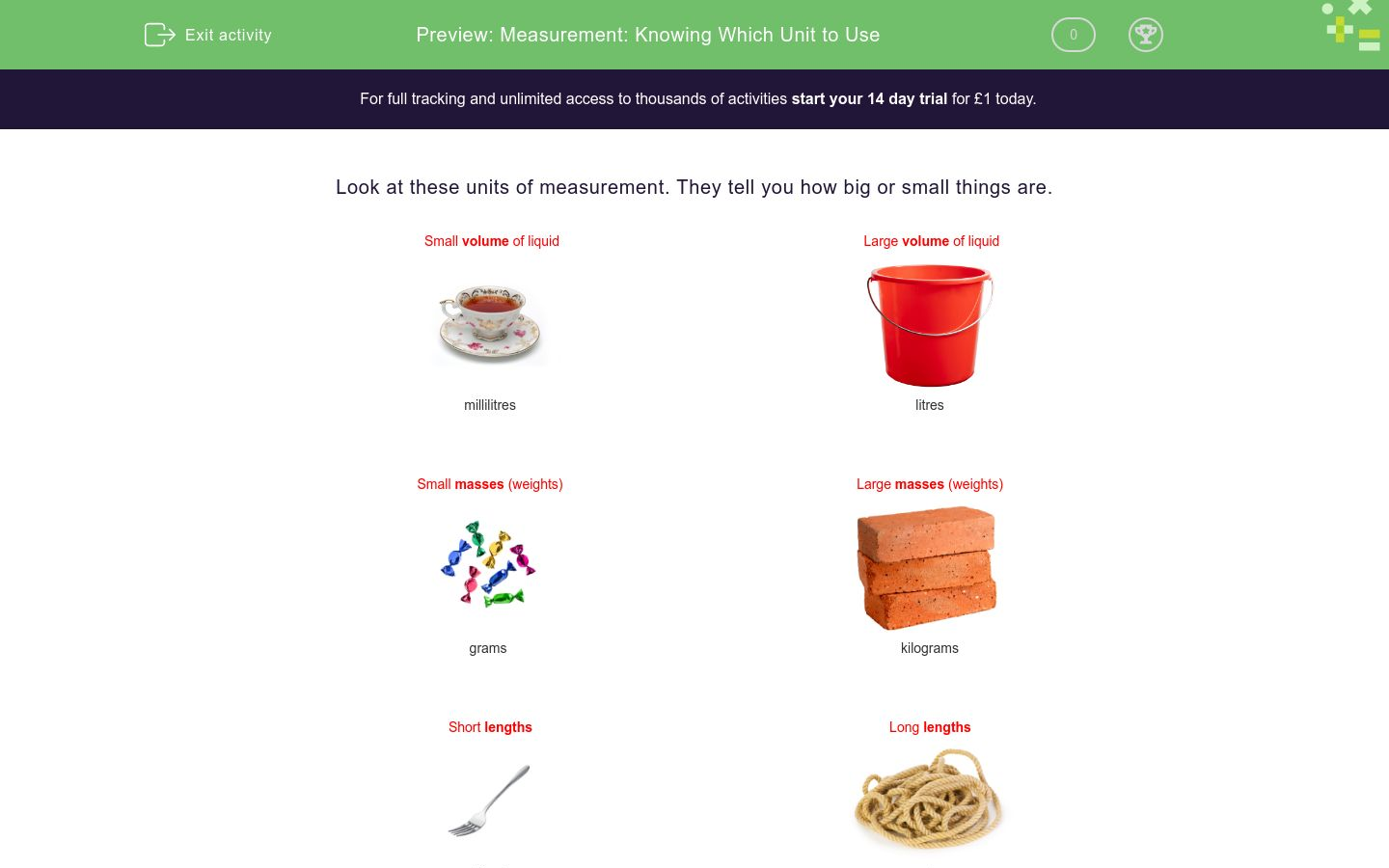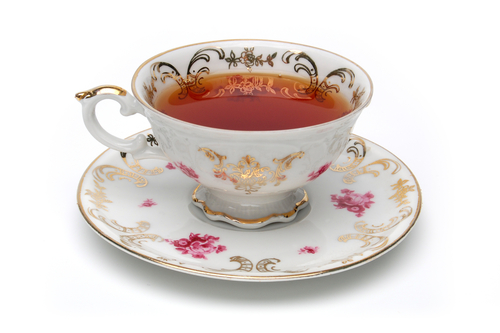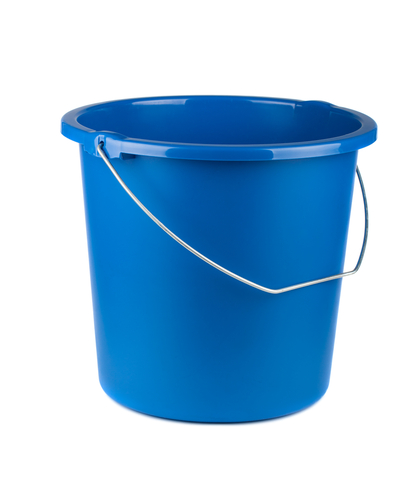# Measurement: Knowing Which Unit to Use

In this worksheet, students choose appropriate units of measurement.Key stage:  KS 1

Curriculum topic:   Measurement

Curriculum subtopic:   Use Standard Units

Difficulty level:### QUESTION 1 of 10

Look at these units of measurement. They tell you how big or small things are.

 Small volume of liquid Large volume of liquidmillilitres litres

 Small masses (weights) Large masses (weights)grams kilograms

 Short lengths Long lengthscentimetres metres

Water inside a bucket is measured in ____________.grams

kilograms

centimetres

metres

millilitres

litres

The mass of a sweet is measured in ____________.grams

kilograms

centimetres

metres

millilitres

litres

This is Fido.

His mass is measured in ____________.grams

kilograms

centimetres

metres

millilitres

litres

The height of this tree is measured in ____________.grams

kilograms

centimetres

metres

millilitres

litres

The mass of an apple is measured in ____________.grams

kilograms

centimetres

metres

millilitres

litres

The mass of this waffle is measured in ____________.grams

kilograms

centimetres

metres

millilitres

litres

The length of this train is measured in ____________.grams

kilograms

centimetres

metres

millilitres

litres

The mass of this car is measured in ____________.grams

kilograms

centimetres

metres

millilitres

litres

The length of this carrot is measured in ____________.grams

kilograms

centimetres

metres

millilitres

litres

The amount of hot chocolate left in this glass is measured in ____________.grams

kilograms

centimetres

metres

millilitres

litres

• Question 1

Water inside a bucket is measured in ____________.litres
EDDIE SAYS
Millilitres measure small volumes.
Litres measure big volumes.
The bucket of water is a big volume.
• Question 2

The mass of a sweet is measured in ____________.grams
EDDIE SAYS
Grams measure a small mass (weight).
Kilograms measure a large mass.
The sweet is a small mass.
• Question 3

This is Fido.

His mass is measured in ____________.kilograms
EDDIE SAYS
Grams are a small mass (weight).
Kilograms are a large mass (weight)
Fido has a large mass.
• Question 4

The height of this tree is measured in ____________.metres
EDDIE SAYS
Centimetres are a shorter than metres.
• Question 5

The mass of an apple is measured in ____________.grams
EDDIE SAYS
Grams have a lighter mass (weight) than kilograms.
Kilograms are heavier than grams.
• Question 6

The mass of this waffle is measured in ____________.grams
EDDIE SAYS
A waffle has a low mass (weight).
It is measured in grams.
• Question 7

The length of this train is measured in ____________.metres
EDDIE SAYS
A train is quite long.
It is measured in metres.
• Question 8

The mass of this car is measured in ____________.kilograms
EDDIE SAYS
A car has a heavy mass (weight). It is measured in kilograms.
• Question 9

The length of this carrot is measured in ____________.centimetres
EDDIE SAYS
A carrot is short.
It is measured in centimetres.
• Question 10

The amount of hot chocolate left in this glass is measured in ____________.millilitres
EDDIE SAYS
The volume (capacity) is very small.
It is measured in millilitres.
---- OR ----

Sign up for a £1 trial so you can track and measure your child's progress on this activity.

### What is EdPlace?

We're your National Curriculum aligned online education content provider helping each child succeed in English, maths and science from year 1 to GCSE. With an EdPlace account you’ll be able to track and measure progress, helping each child achieve their best. We build confidence and attainment by personalising each child’s learning at a level that suits them.

Get started